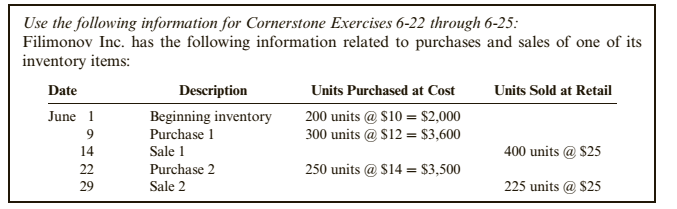Chapter 6, Problem 25CE### Cornerstones of Financial Accounti...

4th Edition
Jay Rich + 1 other
ISBN: 9781337690881

#### Solutions

Chapter
Section### Cornerstones of Financial Accounti...

4th Edition
Jay Rich + 1 other
ISBN: 9781337690881
Textbook Problem
1 views

#Effects of Inventory Costing MethodsRefer to your answers for Filimonov Inc. in Cornerstone Exercises 6-22 through 6-24.Required:1. In a period of rising prices, which inventory costing method produces the highest amount for ending inventory?2. In a period of rising prices, which inventory costing method produces the highest net income?3. In a period of rising prices, which inventory costing method produces the lowest payment for income taxes?4. In a period of rising prices, which inventory method generally produces the most realistic amount for cost of goods sold? For inventory? Would your answer change if inventory prices were decreasing during the period?

To determine

Concept introduction:

FIFO method:

FIFO Stands for First In First Out. Under this method, the units purchased first are assumed to be sold first and cost of goods sold is calculated accordingly. The ending inventory in the method includes the latest units purchased.

LIFO method:

LIFO Stands for Last In First Out. Under this method, the latest units purchased are assumed to be sold first and cost of goods sold is calculated accordingly. The ending inventory in the method includes the oldest units purchased.

Specific identification method:

Under this method the cost of goods sold and ending inventory units are identifiable and the cost is calculated accurately for each unit sold and in the inventory.

Weighted Average method:

Under this method, the cost per unit of the inventory is calculated as weighted average cost per unit and the cost of goods sold and inventory is calculated with the help of weighted average cost per unit.

Requirement 1:

To identify:

The costing method that produces the higher amount of ending inventory in case of rising prices.

Explanation

FIFO Stands for First In First Out. Under this method, the units purchased first are assumed to be sold first and cost of goods sold is calculated accordingly...

To determine

Concept introduction:

FIFO method:

FIFO Stands for First In First Out. Under this method, the units purchased first are assumed to be sold first and cost of goods sold is calculated accordingly. The ending inventory in the method includes the latest units purchased.

LIFO method:

LIFO Stands for Last In First Out. Under this method, the latest units purchased are assumed to be sold first and cost of goods sold is calculated accordingly. The ending inventory in the method includes the oldest units purchased.

Specific identification method:

Under this method the cost of goods sold and ending inventory units are identifiable and the cost is calculated accurately for each unit sold and in the inventory.

Weighted Average method:

Under this method, the cost per unit of the inventory is calculated as weighted average cost per unit and the cost of goods sold and inventory is calculated with the help of weighted average cost per unit.

Requirement 2:

To identify:

The costing method that produces the higher amount of Net income in case of rising prices.

To determine

Concept introduction:

FIFO method:

FIFO Stands for First In First Out. Under this method, the units purchased first are assumed to be sold first and cost of goods sold is calculated accordingly. The ending inventory in the method includes the latest units purchased.

LIFO method:

LIFO Stands for Last In First Out. Under this method, the latest units purchased are assumed to be sold first and cost of goods sold is calculated accordingly. The ending inventory in the method includes the oldest units purchased.

Specific identification method:

Under this method the cost of goods sold and ending inventory units are identifiable and the cost is calculated accurately for each unit sold and in the inventory.

Weighted Average method:

Under this method, the cost per unit of the inventory is calculated as weighted average cost per unit and the cost of goods sold and inventory is calculated with the help of weighted average cost per unit.

Requirement 3:

To identify:

The costing method that produces the lowest payment of income tax in case of rising prices.

To determine

Concept introduction:

FIFO method:

FIFO Stands for First In First Out. Under this method, the units purchased first are assumed to be sold first and cost of goods sold is calculated accordingly. The ending inventory in the method includes the latest units purchased.

LIFO method:

LIFO Stands for Last In First Out. Under this method, the latest units purchased are assumed to be sold first and cost of goods sold is calculated accordingly. The ending inventory in the method includes the oldest units purchased.

Specific identification method:

Under this method the cost of goods sold and ending inventory units are identifiable and the cost is calculated accurately for each unit sold and in the inventory.

Weighted Average method:

Under this method, the cost per unit of the inventory is calculated as weighted average cost per unit and the cost of goods sold and inventory is calculated with the help of weighted average cost per unit.

Requirement 4:

To identify:

The costing method that produces the most realistic amount of cost of goods sold in case of rising prices.

### Still sussing out bartleby?

Check out a sample textbook solution.

See a sample solution

#### The Solution to Your Study Problems

Bartleby provides explanations to thousands of textbook problems written by our experts, many with advanced degrees!

Get Started

#### Find more solutions based on key concepts